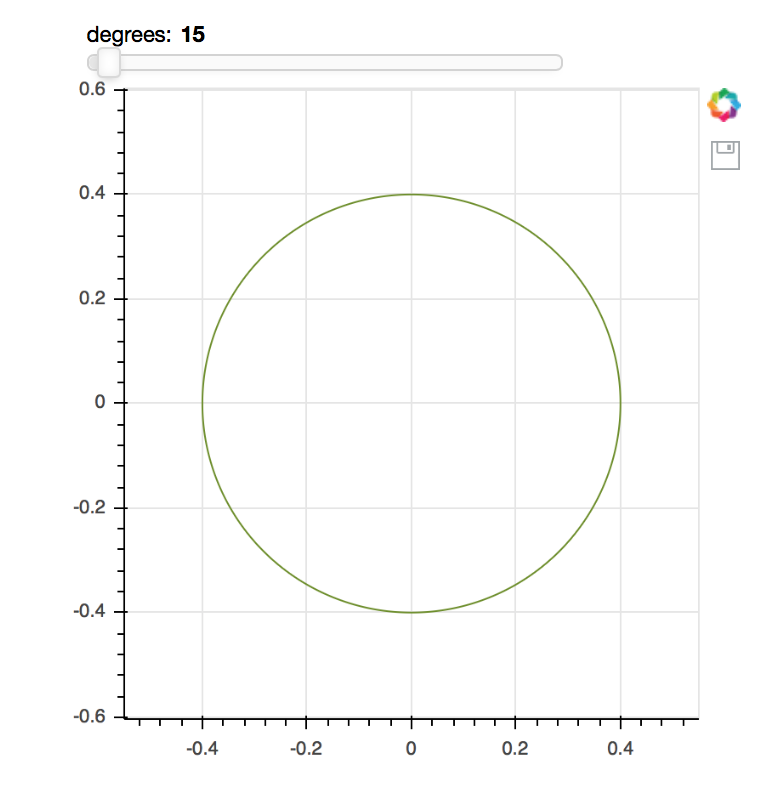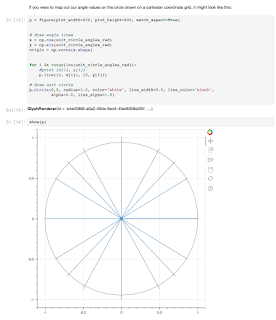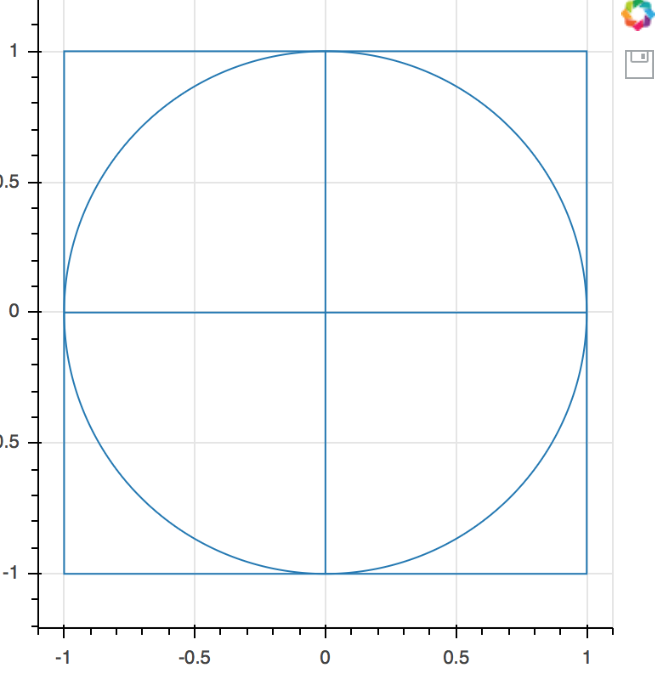# Drawing a unit circle

Pardon the noob question, but all I want to do is draw a unit circle with aspect ratio = 1 (so it looks like a circle and not an ellipse). I’ve been thwarted at every turn.

I’ve tried two basic strategies. First is to create a single point at (0,0) and then specify a radius of 1. Unfortunately, this gives me something that looks circular but doesn’t actually reach all the way up and down to y = +/-1. Second strategy is to plot the points (cos(th), sin(th) ) where th is 0 to 360 degrees. The resulting plot looks elliptical because I can’t get the aspect ratio right.

I don’t know if this is a confounding factor, but I’m including a slider (for something I’ll be doing later) and I don’t know if that’s what’s screwing things up.

The code and results for both strategies are here:

https://vectortronic.github.io/bokeh_scripts

Hi,

As described in the docs:

The match_aspect flag only has effect with the ranges are "auto" DataRange1d ranges. These are the default. In that case Bokeh will adjust the data space range boundaries as necessary to maintain aspect that matches the pixel aspect. However, if you explicitly provide range boundaries, as you have done in your code, Bokeh will not override them.

Thanks

Bryan

···

On Nov 14, 2017, at 12:36, vectortronic <[email protected]> wrote:

Pardon the noob question, but all I want to do is draw a unit circle with aspect ratio = 1 (so it looks like a circle and not an ellipse). I've been thwarted at every turn.

I've tried two basic strategies. First is to create a single point at (0,0) and then specify a radius of 1. Unfortunately, this gives me something that looks circular but doesn't actually reach all the way up and down to y = +/-1. Second strategy is to plot the points (cos(th), sin(th) ) where th is 0 to 360 degrees. The resulting plot looks elliptical because I can't get the aspect ratio right.

I don't know if this is a confounding factor, but I'm including a slider (for something I'll be doing later) and I don't know if that's what's screwing things up.

The code and results for both strategies are here:
https://vectortronic.github.io/bokeh_scripts

--
You received this message because you are subscribed to the Google Groups "Bokeh Discussion - Public" group.
To unsubscribe from this group and stop receiving emails from it, send an email to [email protected].
To post to this group, send email to [email protected].
To view this discussion on the web visit https://groups.google.com/a/continuum.io/d/msgid/bokeh/77daa78c-6774-4d93-b063-1316814c363d%40continuum.io.

With these changes:

``````plot = figure(plot_width=400, plot_height=400, match_aspect=True, tools='save')

``````

We get the following (matched aspect) circle:That said with larger radius values it did not seem to auto-range well. (The circle was outside and surrounding the visible view area.) I’d suggest making a GH issue about improving auto-ranging for circles with radius specified:

``````[https://github.com/bokeh/bokeh/issues](https://github.com/bokeh/bokeh/issues)
``````

Thanks,

Bryan

···

On Nov 14, 2017, at 12:51, Bryan Van de ven [email protected] wrote:

Hi,

As described in the docs:

The match_aspect flag only has effect with the ranges are “auto” DataRange1d ranges. These are the default. In that case Bokeh will adjust the data space range boundaries as necessary to maintain aspect that matches the pixel aspect. However, if you explicitly provide range boundaries, as you have done in your code, Bokeh will not override them.

Thanks

Bryan

On Nov 14, 2017, at 12:36, vectortronic [email protected] wrote:

Pardon the noob question, but all I want to do is draw a unit circle with aspect ratio = 1 (so it looks like a circle and not an ellipse). I’ve been thwarted at every turn.

I’ve tried two basic strategies. First is to create a single point at (0,0) and then specify a radius of 1. Unfortunately, this gives me something that looks circular but doesn’t actually reach all the way up and down to y = +/-1. Second strategy is to plot the points (cos(th), sin(th) ) where th is 0 to 360 degrees. The resulting plot looks elliptical because I can’t get the aspect ratio right.

I don’t know if this is a confounding factor, but I’m including a slider (for something I’ll be doing later) and I don’t know if that’s what’s screwing things up.

The code and results for both strategies are here:
https://vectortronic.github.io/bokeh_scripts

You received this message because you are subscribed to the Google Groups “Bokeh Discussion - Public” group.
To unsubscribe from this group and stop receiving emails from it, send an email to [email protected].
To post to this group, send email to [email protected].
To view this discussion on the web visit https://groups.google.com/a/continuum.io/d/msgid/bokeh/77daa78c-6774-4d93-b063-1316814c363d%40continuum.io.

Ah, great catch. Thanks!

My v2 (where I draw all 360 points) renders perfectly now. Thanks.

···

On Tuesday, November 14, 2017 at 12:58:37 PM UTC-5, Bryan Van de ven wrote:

With these changes:

plot = figure(plot_width=400, plot_height=400, match_aspect=True, tools=‘save’)

We get the following (matched aspect) circle:

That said with larger radius values it did not seem to auto-range well. (The circle was outside and surrounding the visible view area.) I’d suggest making a GH issue about improving auto-ranging for circles with radius specified:

https://github.com/bokeh/bokeh/issues

Thanks,

Bryan

On Nov 14, 2017, at 12:51, Bryan Van de ven [email protected] wrote:

Hi,

As described in the docs:

``````[https://bokeh.pydata.org/en/dev/docs/reference/models/plots.html#bokeh.models.plots.Plot.match_as](https://bokeh.pydata.org/en/dev/docs/reference/models/plots.html#bokeh.models.plots.Plot.match_as)pect
``````

The match_aspect flag only has effect with the ranges are “auto” DataRange1d ranges. These are the default. In that case Bokeh will adjust the data space range boundaries as necessary to maintain aspect that matches the pixel aspect. However, if you explicitly provide range boundaries, as you have done in your code, Bokeh will not override them.

Thanks

Bryan

On Nov 14, 2017, at 12:36, vectortronic [email protected] wrote:

Pardon the noob question, but all I want to do is draw a unit circle with aspect ratio = 1 (so it looks like a circle and not an ellipse). I’ve been thwarted at every turn.

I’ve tried two basic strategies. First is to create a single point at (0,0) and then specify a radius of 1. Unfortunately, this gives me something that looks circular but doesn’t actually reach all the way up and down to y = +/-1. Second strategy is to plot the points (cos(th), sin(th) ) where th is 0 to 360 degrees. The resulting plot looks elliptical because I can’t get the aspect ratio right.

I don’t know if this is a confounding factor, but I’m including a slider (for something I’ll be doing later) and I don’t know if that’s what’s screwing things up.

The code and results for both strategies are here:
https://vectortronic.github.io/bokeh_scripts

You received this message because you are subscribed to the Google Groups “Bokeh Discussion - Public” group.
To unsubscribe from this group and stop receiving emails from it, send an email to [email protected].
To post to this group, send email to [email protected].
To view this discussion on the web visit https://groups.google.com/a/continuum.io/d/msgid/bokeh/77daa78c-6774-4d93-b063-1316814c363d%40continuum.io.

I’m seeing the same problems (strangely, I was trying to get a unit circle drawn today as well). This is what I get:···

On Tuesday, November 14, 2017 at 11:58:37 AM UTC-6, Bryan Van de ven wrote:

With these changes:

plot = figure(plot_width=400, plot_height=400, match_aspect=True, tools=‘save’)

We get the following (matched aspect) circle:

That said with larger radius values it did not seem to auto-range well. (The circle was outside and surrounding the visible view area.) I’d suggest making a GH issue about improving auto-ranging for circles with radius specified:

https://github.com/bokeh/bokeh/issues

Thanks,

Bryan

On Nov 14, 2017, at 12:51, Bryan Van de ven [email protected] wrote:

Hi,

As described in the docs:

``````[https://bokeh.pydata.org/en/dev/docs/reference/models/plots.html#bokeh.models.plots.Plot.match_as](https://bokeh.pydata.org/en/dev/docs/reference/models/plots.html#bokeh.models.plots.Plot.match_as)pect
``````

The match_aspect flag only has effect with the ranges are “auto” DataRange1d ranges. These are the default. In that case Bokeh will adjust the data space range boundaries as necessary to maintain aspect that matches the pixel aspect. However, if you explicitly provide range boundaries, as you have done in your code, Bokeh will not override them.

Thanks

Bryan

On Nov 14, 2017, at 12:36, vectortronic [email protected] wrote:

Pardon the noob question, but all I want to do is draw a unit circle with aspect ratio = 1 (so it looks like a circle and not an ellipse). I’ve been thwarted at every turn.

I’ve tried two basic strategies. First is to create a single point at (0,0) and then specify a radius of 1. Unfortunately, this gives me something that looks circular but doesn’t actually reach all the way up and down to y = +/-1. Second strategy is to plot the points (cos(th), sin(th) ) where th is 0 to 360 degrees. The resulting plot looks elliptical because I can’t get the aspect ratio right.

I don’t know if this is a confounding factor, but I’m including a slider (for something I’ll be doing later) and I don’t know if that’s what’s screwing things up.

The code and results for both strategies are here:
https://vectortronic.github.io/bokeh_scripts

You received this message because you are subscribed to the Google Groups “Bokeh Discussion - Public” group.
To unsubscribe from this group and stop receiving emails from it, send an email to [email protected].
To post to this group, send email to [email protected].
To view this discussion on the web visit https://groups.google.com/a/continuum.io/d/msgid/bokeh/77daa78c-6774-4d93-b063-1316814c363d%40continuum.io.

Travis,

from bokeh.plotting import figure, output_file, show

``````output_file("circle_v1.html")

p = figure(plot_width=400, plot_height=400, match_aspect=True, tools='save')
p.line((0, 1), (0, 0))
p.line((0, -1), (0, 0))
p.line((0, 0), (0, 1))
p.line((0, 0), (0, -1))
p.rect(0, 0, 2, 2, fill_alpha=0)

show(p)
``````

then things work as expected:However, without the rect, I see a result similar to yours. This seems like a bug so I’d suggest a GH issue with details.

Thanks,

Bryan

···

On Nov 14, 2017, at 16:15, [email protected] wrote:

I’m seeing the same problems (strangely, I was trying to get a unit circle drawn today as well). This is what I get:

On Tuesday, November 14, 2017 at 11:58:37 AM UTC-6, Bryan Van de ven wrote:
With these changes:

plot = figure(plot_width=400, plot_height=400, match_aspect=True, tools=‘save’)

We get the following (matched aspect) circle:

That said with larger radius values it did not seem to auto-range well. (The circle was outside and surrounding the visible view area.) I’d suggest making a GH issue about improving auto-ranging for circles with radius specified:

https://github.com/bokeh/bokeh/issues

Thanks,

Bryan

On Nov 14, 2017, at 12:51, Bryan Van de ven [email protected] wrote:

Hi,

As described in the docs:

``````https://bokeh.pydata.org/en/dev/docs/reference/models/plots.html#bokeh.models.plots.Plot.match_aspect
``````

The match_aspect flag only has effect with the ranges are “auto” DataRange1d ranges. These are the default. In that case Bokeh will adjust the data space range boundaries as necessary to maintain aspect that matches the pixel aspect. However, if you explicitly provide range boundaries, as you have done in your code, Bokeh will not override them.

Thanks

Bryan

On Nov 14, 2017, at 12:36, vectortronic [email protected] wrote:

Pardon the noob question, but all I want to do is draw a unit circle with aspect ratio = 1 (so it looks like a circle and not an ellipse). I’ve been thwarted at every turn.

I’ve tried two basic strategies. First is to create a single point at (0,0) and then specify a radius of 1. Unfortunately, this gives me something that looks circular but doesn’t actually reach all the way up and down to y = +/-1. Second strategy is to plot the points (cos(th), sin(th) ) where th is 0 to 360 degrees. The resulting plot looks elliptical because I can’t get the aspect ratio right.

I don’t know if this is a confounding factor, but I’m including a slider (for something I’ll be doing later) and I don’t know if that’s what’s screwing things up.

The code and results for both strategies are here:
https://vectortronic.github.io/bokeh_scripts

You received this message because you are subscribed to the Google Groups “Bokeh Discussion - Public” group.
To unsubscribe from this group and stop receiving emails from it, send an email to [email protected].
To post to this group, send email to [email protected].
To view this discussion on the web visit https://groups.google.com/a/continuum.io/d/msgid/bokeh/77daa78c-6774-4d93-b063-1316814c363d%40continuum.io.

You received this message because you are subscribed to the Google Groups “Bokeh Discussion - Public” group.
To unsubscribe from this group and stop receiving emails from it, send an email to [email protected].
To post to this group, send email to [email protected].
To view this discussion on the web visit https://groups.google.com/a/continuum.io/d/msgid/bokeh/3de804db-9a2a-4d4b-b3f3-95e490fab31b%40continuum.io.

Travis,

from bokeh.plotting import figure, output_file, show

``````output_file("circle_v1.html")

p = figure(plot_width=400, plot_height=400, match_aspect=True, tools='save')
p.line((0, 1), (0, 0))
p.line((0, -1), (0, 0))
p.line((0, 0), (0, 1))
p.line((0, 0), (0, -1))
p.rect(0, 0, 2, 2, fill_alpha=0)

show(p)
``````

then things work as expected:

<Screen Shot 2017-11-14 at 19.34.46.png>

However, without the rect, I see a result similar to yours. This seems like a bug so I’d suggest a GH issue with details.

I’ll file the issue. Thank you very much for the sanity check!

Best,

Travis

···

On Nov 14, 2017, at 7:36 PM, Bryan Van de ven [email protected] wrote:

Thanks,

Bryan

On Nov 14, 2017, at 16:15, [email protected] wrote:

I’m seeing the same problems (strangely, I was trying to get a unit circle drawn today as well). This is what I get:

On Tuesday, November 14, 2017 at 11:58:37 AM UTC-6, Bryan Van de ven wrote:
With these changes:

``````plot = figure(plot_width=400, plot_height=400, match_aspect=True, tools='save')
``````

We get the following (matched aspect) circle:

That said with larger radius values it did not seem to auto-range well. (The circle was outside and surrounding the visible view area.) I’d suggest making a GH issue about improving auto-ranging for circles with radius specified:

``````[https://github.com/bokeh/](https://github.com/bokeh/)bokeh/issues
``````

Thanks,

Bryan

On Nov 14, 2017, at 12:51, Bryan Van de ven <bry…@anaconda.com> wrote:

Hi,

As described in the docs:

``````[https://bokeh.pydata.org/en/dev/docs/reference/models/plots.html#bokeh.models.plots.Plot.match_aspect](https://bokeh.pydata.org/en/dev/docs/reference/models/plots.html#bokeh.models.plots.Plot.match_aspect)
``````

The match_aspect flag only has effect with the ranges are “auto” DataRange1d ranges. These are the default. In that case Bokeh will adjust the data space range boundaries as necessary to maintain aspect that matches the pixel aspect. However, if you explicitly provide range boundaries, as you have done in your code, Bokeh will not override them.

Thanks

Bryan

On Nov 14, 2017, at 12:36, vectortronic <iob…@gmail.com> wrote:

Pardon the noob question, but all I want to do is draw a unit circle with aspect ratio = 1 (so it looks like a circle and not an ellipse). I’ve been thwarted at every turn.

I’ve tried two basic strategies. First is to create a single point at (0,0) and then specify a radius of 1. Unfortunately, this gives me something that looks circular but doesn’t actually reach all the way up and down to y = +/-1. Second strategy is to plot the points (cos(th), sin(th) ) where th is 0 to 360 degrees. The resulting plot looks elliptical because I can’t get the aspect ratio right.

I don’t know if this is a confounding factor, but I’m including a slider (for something I’ll be doing later) and I don’t know if that’s what’s screwing things up.

The code and results for both strategies are here:
https://vectortronic.github.io/bokeh_scripts

You received this message because you are subscribed to the Google Groups “Bokeh Discussion - Public” group.
To unsubscribe from this group and stop receiving emails from it, send an email to bokeh+un…@continuum.io.
To post to this group, send email to bo…@continuum.io.
To view this discussion on the web visit https://groups.google.com/a/continuum.io/d/msgid/bokeh/77daa78c-6774-4d93-b063-1316814c363d%40continuum.io.

You received this message because you are subscribed to the Google Groups “Bokeh Discussion - Public” group.
To unsubscribe from this group and stop receiving emails from it, send an email to [email protected].
To post to this group, send email to [email protected].
To view this discussion on the web visit https://groups.google.com/a/continuum.io/d/msgid/bokeh/3de804db-9a2a-4d4b-b3f3-95e490fab31b%40continuum.io.